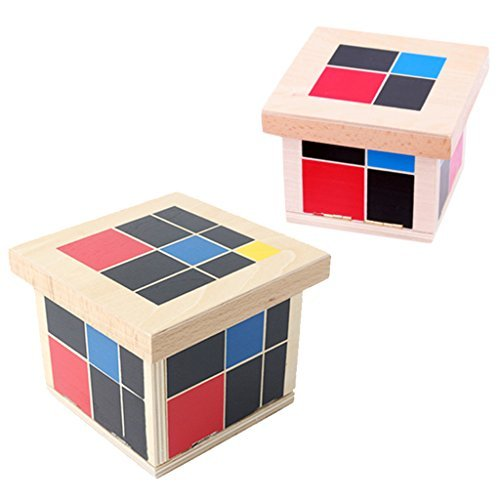# MonkeyJack Wooden Montessori Mathematics Material - Trinomial and Binomial Cube

MonkeyJack Wooden Montessori Mathematics Material - Trinomial and Binomial Cube by MonkeyJack at CACCB. MPN: b8fa5e4c2f8701a144f7e2fbe980c899. Hurry! Limited time offer. Offer valid only while supplies last. Description:Wooden binomial and trinomial cube kids childrens learning algebra & maths educational toysMaterial: BeechwoodBinomial Cube is composed of 8 wooden blocks which fit together in a binomial pattern, forming a concrete representation of the algebraic formula (a+b)3=a3+3a2b+3ab2+b3 Trinomial Cube is composed of 27 wooden blocks which fit together in a trinomal pattern, forming a concrete representation of the algebraic formula (a+b+c)3=a3+b3+c3+3a2b+3ab2+3b2c+3bc2+3a2c+3ac2+6abc. Each Fa4.5 out of 5 stars with 36 reviews
Condition: New
Availability: In Stock
\$35.83

Quantity:

## Product Description & Reviews

Description:Wooden binomial and trinomial cube kids childrens learning algebra & maths educational toysMaterial: BeechwoodBinomial Cube is composed of 8 wooden blocks which fit together in a binomial pattern, forming a concrete representation of the algebraic formula (a+b)3=a3+3a2b+3ab2+b3 Trinomial Cube is composed of 27 wooden blocks which fit together in a trinomal pattern, forming a concrete representation of the algebraic formula (a+b+c)3=a3+b3+c3+3a2b+3ab2+3b2c+3bc2+3a2c+3ac2+6abc. Each Face that "a2" is red, "b2" is blue, "c2" is yellow and all other faces are black The factors of the equation are represented by the color coded cubes and prisms. Younger children can explore the binomial cube as a sensorial activity of visual discrimination of color and form. This can serve as an indirect preparation for algebra and mathematicsExcellent homeschool or classroom educational materialSize Chart:Binomial cube size: Approx. 9.5 x 9.5 x 8.5cm/ 3.74 x 3.74 x 3.34inchTrinomial cube size: Approx. 13.2 x 13.2 x 10.8cm/ 5.19 x 5.19 x 4.25inchPackage Includes:1 set of binomial cube1 set of trinomial cube

## Features & Highlights

• Wooden binomial and trinomial cube kids childrens learning algebra & maths educational toys
• Material: Beechwood
• Binomial Cube is composed of 8 wooden blocks which fit together in a binomial pattern, forming a concrete representation of the algebraic formula (a+b)3=a3+3a2b+3ab2+b3
• Trinomial Cube is composed of 27 wooden blocks which fit together in a trinomal pattern, forming a concrete representation of the algebraic formula (a+b+c)3=a3+b3+c3+3a2b+3ab2+3b2c+3bc2+3a2c+3ac2+6abc. Each Face that "a2" is red, "b2" is blue, "c2" is yellow and all other faces are black
• The factors of the equation are represented by the color coded cubes and prisms. Younger children can explore the binomial cube as a sensorial activity of visual discrimination of color and form. This can serve as an indirect preparation for algebra and mathematics

Brand:
MonkeyJack
Manufacturer:
MonkeyJack
Category:
Counting & Math Toys
MPN:
b8fa5e4c2f8701a144f7e2fbe980c899
UPC:
704583966059
Part Number:
b8fa5e4c2f8701a144f7e2fbe980c899
Size:
9.5cm, 13.2cm
Publisher:
MonkeyJack
Binding:
Toy
Package Weight:
2 pounds
Package Size:
6 x 6 x 6 inches

First Name:
Last Name:

## Related Best Sellers

mpn: JMS021 & JMS022, ean: 9780740325311, isbn: 0740325310,
Horizons 2nd Grade Math Student Book 1 contains fun, entertaining lessons that include: counting and writing numbers by 1s to 100-999; using tally marks; recognizing ordinal numbers to 100, less than, greater than, or equal to; adding and subtraction...

mpn: LER0383, ean: 0765023803839,
Your students will practice number recognition, addition, and subtraction with this fast-paced game. Includes 2 number cubes, 1 operation cube, 28 cardboard markers, and activity guide.Numbers and colors on an oversized mat with giant dice. Students ...

mpn: LER0935, ean: 0858160437651,
This large number line format lets kids interact with the most common number line format in the curriculum! Numerals are color-coded; blue evens, red odds and black zero. Mat measures 22'L x 1'H.Combine number learning with movement with this interac...

mpn: LER1766, ean: 0765023817669,
Introduce and engage children in composing 2-D shapes. All pieces are relational and translucent. This set of 408 pieces includes 14 different shapes in 7 colors (2 different squares, rectangle, 5 different triangles, hexagon, trapezoid, rhombus, cir...